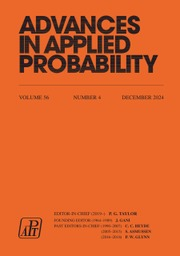Home
Hostname: page-component-79b67bcb76-tlg78 Total loading time: 0.804 Render date: 2021-05-13T03:32:43.105Z Has data issue: true Feature Flags: { "shouldUseShareProductTool": true, "shouldUseHypothesis": true, "isUnsiloEnabled": true, "metricsAbstractViews": false, "figures": false, "newCiteModal": false, "newCitedByModal": true, "newEcommerce": true }Advances in Applied Probability

# On the Dual Relationship Between Markov Chains of GI/M/1 and M/G/1 Type

Published online by Cambridge University Press:  01 July 2016

## Abstract

In 1990, Ramaswami proved that, given a Markov renewal process of M/G/1 type, it is possible to construct a Markov renewal process of GI/M/1 type such that the matrix transforms G (z, s) for the M/G/1-type process and R (z, s) for the GI/M/1-type process satisfy a duality relationship. In his 1996 PhD thesis, Bright used time reversal arguments to show that it is possible to define a different dual for positive-recurrent and transient processes of M/G/1 type and GI/M/1 type. Here we compare the properties of the Ramaswami and Bright dual processes and show that the Bright dual has desirable properties that can be exploited in the design of algorithms for the analysis of Markov chains of GI/M/1 type and M/G/1 type.

## MSC classification

Type
General Applied Probability
Information
Advances in Applied Probability , March 2010 , pp. 210 - 225

## References

Akar, N. and Sohraby, K. (1997). An invariant subspace approach in M/G/1 and G/M/1 type Markov chains. Commun. Statist. Stoch. Models 13, 381416.CrossRefGoogle Scholar
Asmussen, S. (1989). Aspects of matrix Wiener–Hopf factorisation in applied probability. Math. Scientist 14, 101116.Google Scholar
Asmussen, S. and Ramaswami, V. (1990). Probabilistic interpretations of some duality results for the matrix paradigms in queueing theory. Commun. Statist. Stoch. Models 6, 715733.CrossRefGoogle Scholar
Bini, D. A. and Meini, B. (1995). On cyclic reduction applied to a class of Toeplitz-like matrices arising in queueing problems. In Computations with Markov Chains, ed. Stewart, W. J., Kluwer, Boston. pp. 2128.CrossRefGoogle Scholar
Bini, D. A, Latouche, G. and Meini, B. (2005). Numerical Methods for Structured Markov Chains. Oxford University Press.CrossRefGoogle Scholar
Bini, D.A., Meini, B. and Ramaswami, V. (2008). A probabilistic interpretation of cyclic reduction and its relationships with logarithmic reduction. Calcolo 45, 207216.CrossRefGoogle Scholar
Bini, D. A., Meini, B., Steffé, S. and Van Houdt, B. (2006). Structured Markov chains solver: software tools. In Proc. SMCTools Workshop (Pisa, 2006; ACM Internat. Conf. Proc. Ser. 201), ACM Press, New York.Google Scholar
Bright, L. (1996). Matrix-analytic methods in applied probability. , University of Adelaide.Google Scholar
Falkenberg, E. (1994). On the asymptotic behaviour of the stationary distribution of Markov chains of M/G/1-type. Commun. Statist. Stoch. Models 10, 7597.CrossRefGoogle Scholar
Gail, H. R., Hantler, S. L. and Taylor, B. A. (1994). Solutions of the basic matrix equation for M/G/1 and G/M/1 type Markov chains. Commun. Statist. Stoch. Models 10, 144.CrossRefGoogle Scholar
Gail, H. R., Hantler, S. L. and Taylor, B. A. (1996). Spectral analysis of M/G/1 and G/M/1 type Markov chains. Adv. Appl. Prob. 28, 114165.CrossRefGoogle Scholar
Gail, H. R., Hantler, S. L. and Taylor, B. A. (1998). Matrix-geometric invariant measures for G/M/1 type Markov chains. Commun. Statist. Stoch. Models 14, 537569.CrossRefGoogle Scholar
Kelly, F. P. (1983). Invariant measures and the q-matrix. In Probability, Statistics and Analysis (London Math. Soc. Lecture Notes 79), eds Kingman, J. F. C. and Reuter, G. E. H., Cambridge University Press, pp. 143160.CrossRefGoogle Scholar
Latouche, G. (1993). Algorithms for infinite Markov chains with repeating columns. In Linear Algebra, Markov Chains, and Queueing Models (IMA Vol. Math. Appl. 48), Springer, New York, pp. 231265.CrossRefGoogle Scholar
Latouche, G. (1994). Newton's iteration for non-linear equations in Markov chains. IMA J. Numer. Anal. 14, 583598.CrossRefGoogle Scholar
Latouche, G. and Ramaswami, V. (1993). A logarithmic reduction algorithm for quasi-birth-and-death processes. J. Appl. Prob. 30, 650674.CrossRefGoogle Scholar
Latouche, G. and Ramaswami, V. (1999). Introduction to Matrix Analytic Methods in Stochastic Modeling. Society for Industrial and Applied Mathematics, Philadelphia, PA.CrossRefGoogle Scholar
Neuts, M. F. (1978). Markov chains with applications in queueing theory, which have a matrix-geometric invariant probability vector. Adv. Appl. Prob. 10, 185212.CrossRefGoogle Scholar
Neuts, M. F. (1981). Matrix-Geometric Solutions in Stochastic Models. John Hopkins University Press, Baltimore, MD.Google Scholar
Neuts, M. F. (1989). Structured Stochastic Matrices of M/G/1 and Their Applications. Marcel Dekker, New York.Google Scholar
Ramaswami, V. (1990). A duality theorem for the matrix paradigm in queueing theory. Commun. Statist. Stoch. Models 6, 151161.CrossRefGoogle ScholarYou have Access

# Send article to Kindle

Note you can select to send to either the @free.kindle.com or @kindle.com variations. ‘@free.kindle.com’ emails are free but can only be sent to your device when it is connected to wi-fi. ‘@kindle.com’ emails can be delivered even when you are not connected to wi-fi, but note that service fees apply.

Find out more about the Kindle Personal Document Service.

On the Dual Relationship Between Markov Chains of GI/M/1 and M/G/1 Type
Available formats
×

# Send article to Dropbox

To send this article to your Dropbox account, please select one or more formats and confirm that you agree to abide by our usage policies. If this is the first time you use this feature, you will be asked to authorise Cambridge Core to connect with your <service> account. Find out more about sending content to Dropbox.

On the Dual Relationship Between Markov Chains of GI/M/1 and M/G/1 Type
Available formats
×

# Send article to Google Drive

To send this article to your Google Drive account, please select one or more formats and confirm that you agree to abide by our usage policies. If this is the first time you use this feature, you will be asked to authorise Cambridge Core to connect with your <service> account. Find out more about sending content to Google Drive.

On the Dual Relationship Between Markov Chains of GI/M/1 and M/G/1 Type
Available formats
×
×